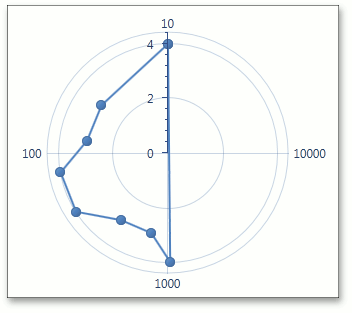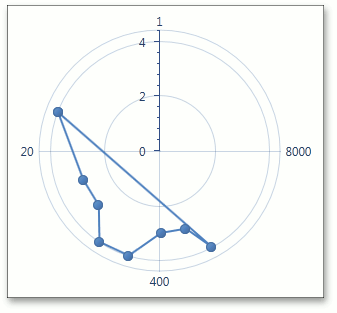Gets or sets a value specifying a logarithmic base when the RadarAxisX2D.Logarithmic property is enabled.

Namespace: DevExpress.Xpf.Charts

Assembly: DevExpress.Xpf.Charts.v21.2.dll

## Declaration

``public double LogarithmicBase { get; set; }``

## Property Value

Type Description
Double

A Double value which is the logarithmic base.

## Remarks

If a chart’s series have their Series.ArgumentScaleType property set to ScaleType.Numerical, you can use the RadarAxisX2D.Logarithmic property to specify whether the logarithmic scale should be used to display axis values. When it is set to true, it is possible to define a logarithmic base value via the LogarithmicBase property.

Via the LogarithmicBase property, you can define a base value as a logarithm, which will be used to calculate the axis values.

The following images demonstrate how the LogarithmicBase property works.

LogarithmicBase = 10

LogarithmicBase = 20

\